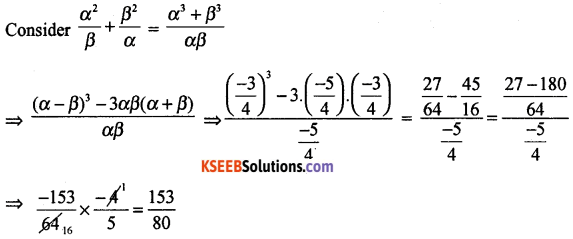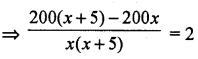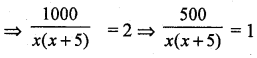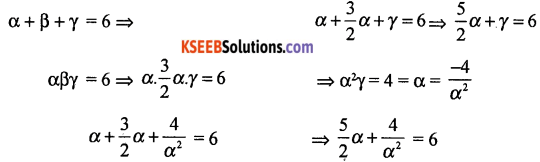# 1st PUC Basic Maths Question Bank Chapter 6 Theory of Equations

Students can Download Basic Maths Chapter 6 Theory of Equations Questions and Answers, Notes Pdf, 1st PUC Basic Maths Question Bank with Answers helps you to revise the complete Karnataka State Board Syllabus and score more marks in your examinations.

## Karnataka 1st PUC Basic Maths Question Bank Chapter 6 Theory of Equations

Question 1.
Find the nature of roots of the equation 3x2 – 4x + 9 = 0
Nature of roots depends on b2 – 4ac
⇒ a = 3, b = – 4, c = 9.
∴ b2 – 4ac = (-4)2 – 4 . (3) . 9 = 16 – 108 = – 92 < 0
∴ The roots are imaginary.

Question 2.
If a and B are the roots of 4x2 + 3x – 5 = 0. Find the value of $$\frac{\alpha^{2}}{\beta}+\frac{\beta^{2}}{\alpha}$$
a = 4, b = 3, c = – 5
Sum of roots = α + β = $$\frac{-b}{a}=\frac{-3}{4}$$
Product of roots = αβ = $$\frac{c}{a}=\frac{-5}{4}$$Question 3.
Find three consecutive even natural numbers, such that the sum of their squares is 200.
Let the required even numbers be x, x + 2, x + 4
By data x2 + (x + 2)2 + (x + 4)2 = 200
⇒ x2 + x2 + 4x + 4 + x2 + 8x + 16 – 200 = 0
3x2 + 12x – 180 = 0 ⇒ x2 + 4x – 60 = 0
⇒ (x + 10)(x – 6) =0
we require +ve the integers ∴ x = 6
⇒ 6,8, 10 be the numbers.

Question 4.
A piece of cloth costs Rs. 200. If the piece were 5mts longer and each meter of cloth costed Rs. 2 less, the cost of piece would have remained unchanged. How long is the piece and what is its original rate per meter.
Let x be the length of the cloth, its cost = 200
∴ Rate of cloth per meter = $$\frac{200}{x}$$
Increased length of piece of cloth = (x + 5) mts
Now rate of cloth per meter = $$\frac{200}{21+5}$$
By date, old rate cloth – new rate of cloth = Rs 2.⇒ x2 + 5x – 500 = 0
⇒ x2 + 25x – 20x – 500 = 0
⇒ x(x + 25) – 20(x + 25) = 0
⇒ x = 20 or -25 (neglected) [∴ x = 20mts]
length of cloth = 20 mts, rate per metre = Rs. 10.

Question 5.
Solve : x3 – 6x2 + 11x – 6 = 0. since that ratio of two roots is 2 : 3
By data α : β = 2 : 3
⇒ $$\frac{\alpha}{\beta}=\frac{2}{3}$$ = α = 2β
from the given equation,⇒ 5α3 – 12α2 + 8 = 0
solving α = 2, ∴ α = 1
∴ The roots are 1,2,3Question 6.
Solve by synthetic division that it has atleast one integral root between -3 and 3 for the equation x4 – 9x2 + 4x + 12 = 0q